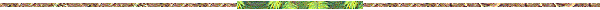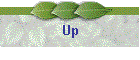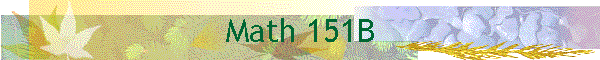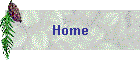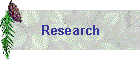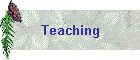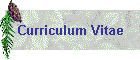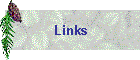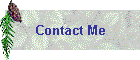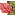Course: Math 151B, Applied Numerical MethodsText: Burden and Faires, Numerical Analysis, 7th ed., Brooks/ColeSchedule of Lectures: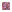Section 5.1: Background for programming projects, introduction to the solution of initial value problemsSection 5.2: Derivations of Euler's method, definition of convergenceSection 5.2-5.3: Error bounds and asymptotic error estimate for Euler's method, local truncation error, global errorSection 5.2-5.4: Convergence proof for Euler's method, derivation of Runge-kutta methodsSection 5.4: Runge-Kutta methods cont, derivation of the general second order Runge-Kutta methodsSection AS: Timestep estimation, model problem analysis, intervals of absolute stabilitySection AS: Timestep estimation for general equationsSection 5.11: Implicit methods (Trapezoidal rule, Backward Euler), comparison of ODE methodsSection 5.11: Implicit methods, solving the implicit equations, stiff differential equationsSection 5.6: Overview of multistep methodsSection 5.9: Numerical methods for systems of ODE'sSection 5.9: Results on numerical methods for systems, convergence results, error bounds, asymptotic error estimates, regions of absolute stability.Section 11.3: Two point boundary values problems, finite difference approximationSection 7.1 11.3: Review of vector and matrix norms, error estimates for linear two-point boundary value problemSection 11.4: Programming consideration for two point boundary value problemsSection 7.3: Iterative methods for the solution of linear systems of equations, Gauss-Jacobi methodSection 7.3: Iterative methods cont, Gauss-Seidel methd, error analysis for iterative methodsSection 7.1-4: Error analysis for iterative methods cont, relationship between error and the residual, stopping criterionSection 7.3-4: Convergence results for iterative methods, condition numberSection 8.1: Discrete least squares approximation, construction of the normal equationsSection 8.1, DLS: Derivation of the normal equations,  matrix/vector formulation of the discrete least squares problemSection DLS: Using the QR decomposition to solve normal equations, relation of QR to Gram-Schmidt.Section 8.5: Introduction to discrete Fourier approximationSection 8.5: Fourier approximation cont, complex form of the discrete Fourier approximationSection 8.6: The fast Fourier transform